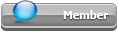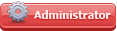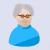## Featured Articles

Check out the latest featured articles.## File Library3# Pressure Change Of Air In A Vessel

air ideal gas law pressure volume

10 replies to this topic
|

### #1panagiotis

panagiotis

Gold Member

•• Members
•• 87 posts

Posted 05 March 2022 - 05:22 AM

Dear all,

I would like to ask you a question, which seems to be simple, but it puzzles me.

I have a vessel that is filled with air at pressure p1 and V1, which V1 is also the volume of the vessel.

Then the pressure in the vessel reduces because the amount of air is leaving the vessel. And the pressure reduces to p2.

I have several values of pressures, while the vessel is "emptying".

I want to use these pressure measurements to calculate the volume of the air.

I have assumed that ideal gas can be applied.

if we assume that I have the below pressures:

p1= known, V1=known

p2=known---------->V2?

p3=known--------->V3?

p4=known-----------> V3?

(pressures are in barg and volume are in m3)

How I can really solve this very simple problem? Do I need to consider the standard conditions?

Let me a bit more clear:

I start with the known values p1, V1 (which is also the volume of the vessel)

NV2=(P2+1,101325)*V1/1,01325     (NV2 is normal volume)

NV3=(P3+1,101325)*V1/1,101325 (NV3 is normal volume)

Is this approach correct?

Is it correct to consider that the volume of air every time will be the volume of the container. And that the normal volume of air changes?

Edited by panagiotis, 05 March 2022 - 05:31 AM.

### #2breizh

breizh

Gold Member

••• 5,421 posts

Posted 05 March 2022 - 06:47 AM

Hi,

Yes the volume is always the volume of the container , the change is about the mass within this volume .

mass = Volume container * density of air .

density of air (P,T) = Molecular weight  air  / molar volume air = Mw/v with  v= Z*R*T/P

Good luck

Breizh

### #3Pilesar

Pilesar

Gold Member

•• Members
•• 967 posts

Posted 05 March 2022 - 07:41 AM

For purposes of your question, we are ignoring containers like latex balloons which can have very large changes in volume. If a vessel is made with stiff material like steel or glass then the vessel volume is usually assumed to be constant since there will only be relatively small changes in volume due to temperature and pressure. In a constant volume vessel, V1 = V2 = V3.

For gas in a constant volume container, the number of molecules, the pressure, and the temperature are related. Keeping the temperature fixed results in the pressure being higher when the vessel contains more molecules. PV=nRT is the general relationship between molecules, pressure, volume, and temperature for gases.

For a fixed volume at a specific pressure and temperature, the number of molecules of gas can be calculated. Because the number of molecules is very large, another way of describing the quantity of molecules is to ask 'If I took this fixed quantity of molecules and changed the pressure and temperature to standard conditions, what would be the volume of this fixed quantity of molecules?' The answer is the standard volume of gas. This means that although the volume of the vessel is assumed fixed, the standard volume of gas inside the vessel is related to the pressure and temperature of the gas.

Standard volume is used as a measurement of a quantity of gas molecules and can be converted directly to moles of gas using a simple conversion factor.

### #4panagiotis

panagiotis

Gold Member

•• Members
•• 87 posts

Posted 05 March 2022 - 07:44 PM

Pilesar,

First of all, thank you for the detailed answer.
Before I comment on your answer, I would like to ask you one more question no one of the different ideal gas law formulas (Boyles, Charles, Gay-Lussac, etc) are applicable to this problem of vessel emptying?

Regarding your answer, I have understood (please confirm) that the idea of standard volume is a way to find a correlation at each pressure measurement. We can see that the normal volume in each pressure (which reduces, while the vessel is emptying)  reduces.
The assumptions for this problem are:
V1=V2=V3=Vvessel
Standard conditions (p=1,01325 and T=15oC)
T inside the vessel constant

Now, if I go back to the equations and take also into account the correction for temperature and the standard conditions, then I will get
NV2=(p2*V*T)/(1,101325*15)
NV3=(p3*V*T)/(1,101325*15)
If I know that to empty this vessel I need time t1, then I can calculate the standard volumetric flow (Q stand), which will be
Qstand=(NV2+NV3+....)/t1.
If I want to calculate the actual volumetric flow, what do I need to do?

Edited by panagiotis, 05 March 2022 - 08:36 PM.

### #5latexman

latexman

Gold Member

••• 1,335 posts

Posted 05 March 2022 - 10:02 PM

PV=nRT

n=PV/RT

dn/dt = V/RT x dP/dt

### #6Bobby Strain

Bobby Strain

Gold Member

•• Members
•• 3,206 posts

Posted 05 March 2022 - 11:39 PM

Yes, it is as simple as Latexman explained. And, that is how you should have started. You know all this stuff as a Chemical Engineer.

Bobby

### #7panagiotis

panagiotis

Gold Member

•• Members
•• 87 posts

Posted 06 March 2022 - 05:03 AM

This equation can give me the number of moles. And the volumetric flow will be Q=((ninitial-nfinal)*Mr)/density of air

Could you answer me something else, what is the benefit of expressing flows in standard volumetric flows?

Edited by panagiotis, 06 March 2022 - 05:33 AM.

### #8Pilesar

Pilesar

Gold Member

•• Members
•• 967 posts

Posted 06 March 2022 - 06:42 AM

Gas standard volumes are a molar measurement. Even though the word 'volume' is in the term, it is a molar measurement. This is why you do not find standard volume terms in a conversion factor table for volume. However, you can convert gas standard volumes directly to moles with a simple conversion factor.

### #9breizh

breizh

Gold Member

••• 5,421 posts

Posted 06 March 2022 - 07:33 AM

Hi,

To clarify let you study the document attached , it should help you .

To avoid mistakes better to refer to mass flow rate , this is my view .

Good luck

Breizh

### #10panagiotis

panagiotis

Gold Member

•• Members
•• 87 posts

Posted 06 March 2022 - 11:47 AM

Bobby and Latexman,

the question you are mentioning is not that clear, to be honest with you.

You do not mention anything about volumetric flows, conditions, etc.

Could you please explain it a bit more?

### #11breizh

breizh

Gold Member

••• 5,421 posts

Posted 06 March 2022 - 07:38 PM

Hi,

To Panagiotis and other members ,

another great resource to support your work :

https://www.cagi.org...Air-System.aspx

Good luck

Breizh

### Similar Topics